Скачать презентацию TX-1037 Mathematical Techniques for Managers Lecture 5

eb7b6ee8a7f29297bee5b1687830e20b.ppt

• Количество слайдов: 23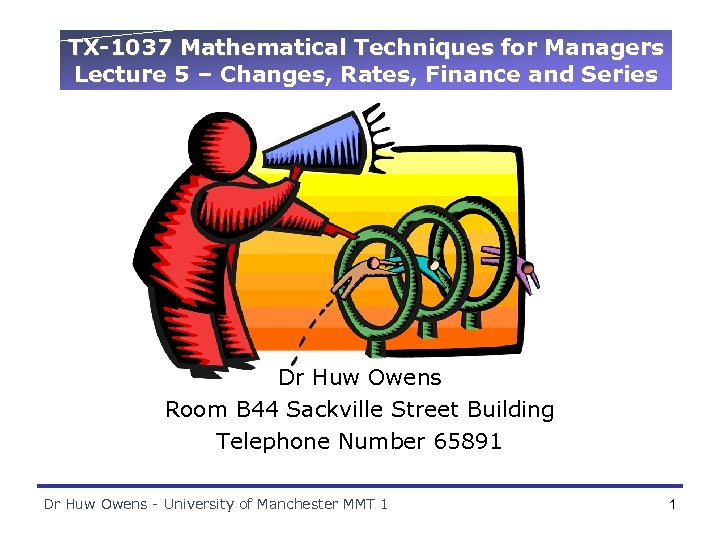TX-1037 Mathematical Techniques for Managers Lecture 5 – Changes, Rates, Finance and Series Dr Huw Owens Room B 44 Sackville Street Building Telephone Number 65891 Dr Huw Owens - University of Manchester MMT 1 1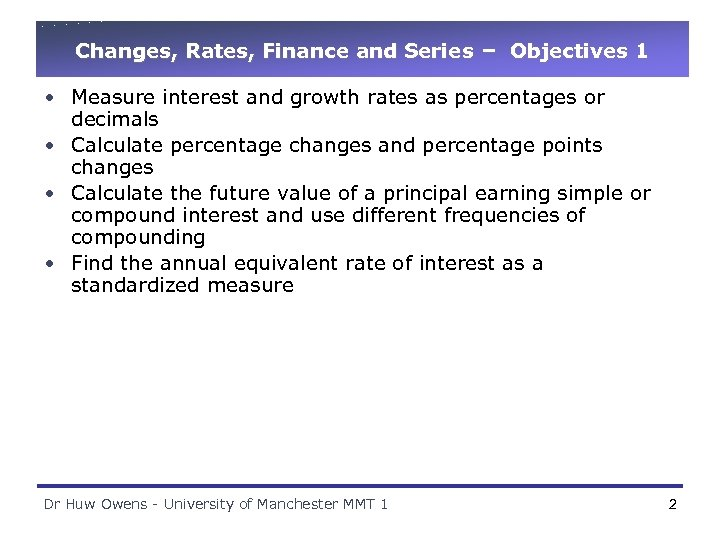Changes, Rates, Finance and Series – Objectives 1 • Measure interest and growth rates as percentages or decimals • Calculate percentage changes and percentage points changes • Calculate the future value of a principal earning simple or compound interest and use different frequencies of compounding • Find the annual equivalent rate of interest as a standardized measure Dr Huw Owens - University of Manchester MMT 1 2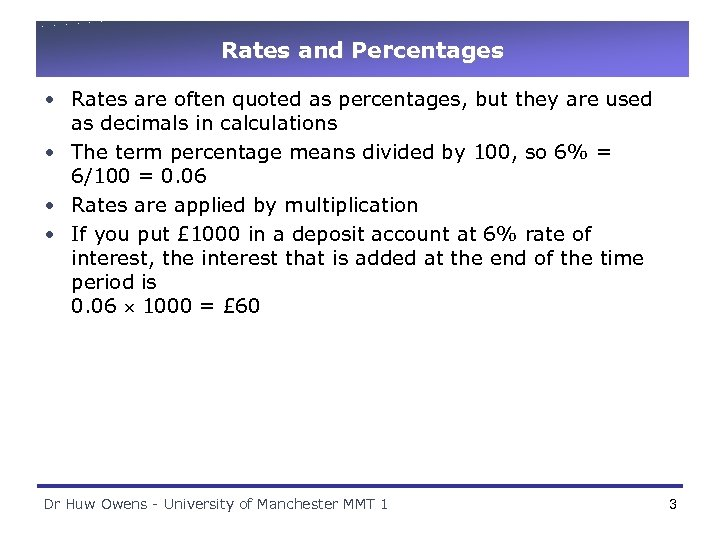Rates and Percentages • Rates are often quoted as percentages, but they are used as decimals in calculations • The term percentage means divided by 100, so 6% = 6/100 = 0. 06 • Rates are applied by multiplication • If you put £ 1000 in a deposit account at 6% rate of interest, the interest that is added at the end of the time period is 0. 06 1000 = £ 60 Dr Huw Owens - University of Manchester MMT 1 3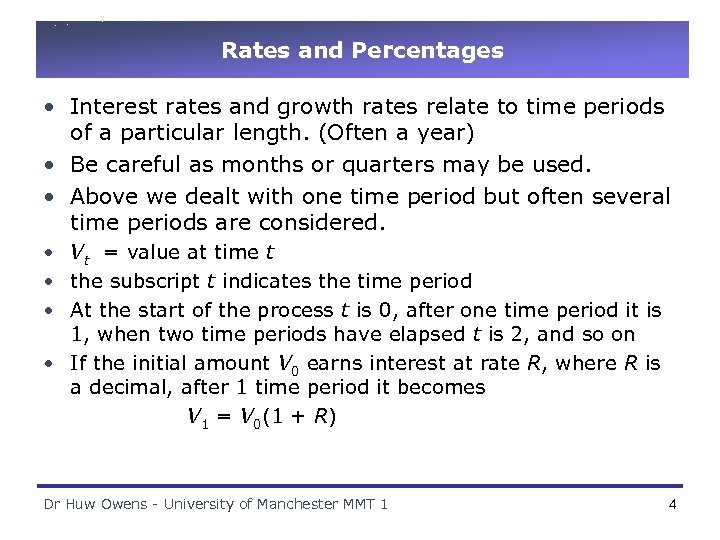Rates and Percentages • Interest rates and growth rates relate to time periods of a particular length. (Often a year) • Be careful as months or quarters may be used. • Above we dealt with one time period but often several time periods are considered. • Vt = value at time t • the subscript t indicates the time period • At the start of the process t is 0, after one time period it is 1, when two time periods have elapsed t is 2, and so on • If the initial amount V 0 earns interest at rate R, where R is a decimal, after 1 time period it becomes V 1 = V 0(1 + R) Dr Huw Owens - University of Manchester MMT 1 4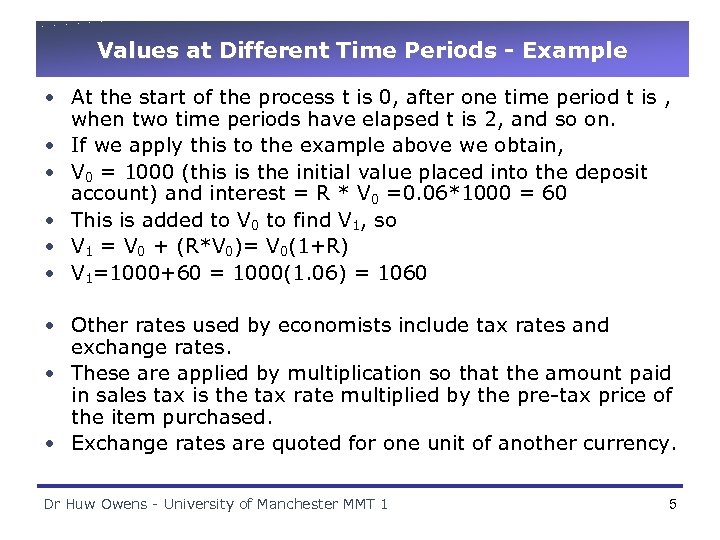Values at Different Time Periods - Example • At the start of the process t is 0, after one time period t is , when two time periods have elapsed t is 2, and so on. • If we apply this to the example above we obtain, • V 0 = 1000 (this is the initial value placed into the deposit account) and interest = R * V 0 =0. 06*1000 = 60 • This is added to V 0 to find V 1, so • V 1 = V 0 + (R*V 0)= V 0(1+R) • V 1=1000+60 = 1000(1. 06) = 1060 • Other rates used by economists include tax rates and exchange rates. • These are applied by multiplication so that the amount paid in sales tax is the tax rate multiplied by the pre-tax price of the item purchased. • Exchange rates are quoted for one unit of another currency. Dr Huw Owens - University of Manchester MMT 1 5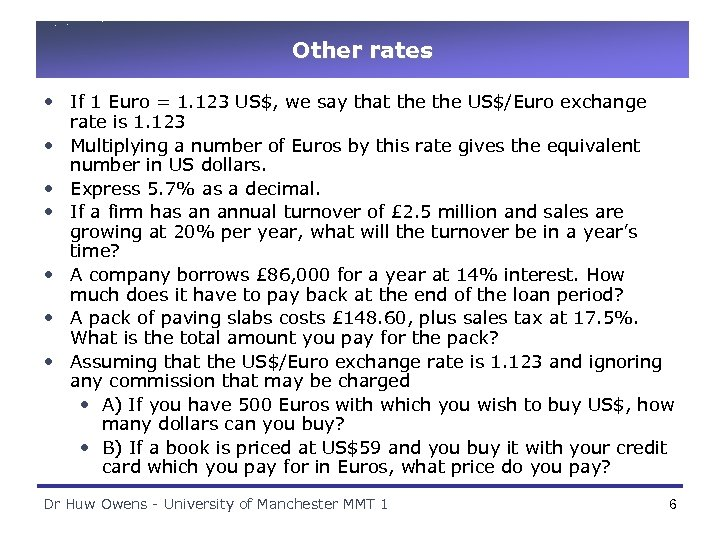Other rates • If 1 Euro = 1. 123 US\$, we say that the US\$/Euro exchange rate is 1. 123 • Multiplying a number of Euros by this rate gives the equivalent number in US dollars. • Express 5. 7% as a decimal. • If a firm has an annual turnover of £ 2. 5 million and sales are growing at 20% per year, what will the turnover be in a year’s time? • A company borrows £ 86, 000 for a year at 14% interest. How much does it have to pay back at the end of the loan period? • A pack of paving slabs costs £ 148. 60, plus sales tax at 17. 5%. What is the total amount you pay for the pack? • Assuming that the US\$/Euro exchange rate is 1. 123 and ignoring any commission that may be charged • A) If you have 500 Euros with which you wish to buy US\$, how many dollars can you buy? • B) If a book is priced at US\$59 and you buy it with your credit card which you pay for in Euros, what price do you pay? Dr Huw Owens - University of Manchester MMT 1 6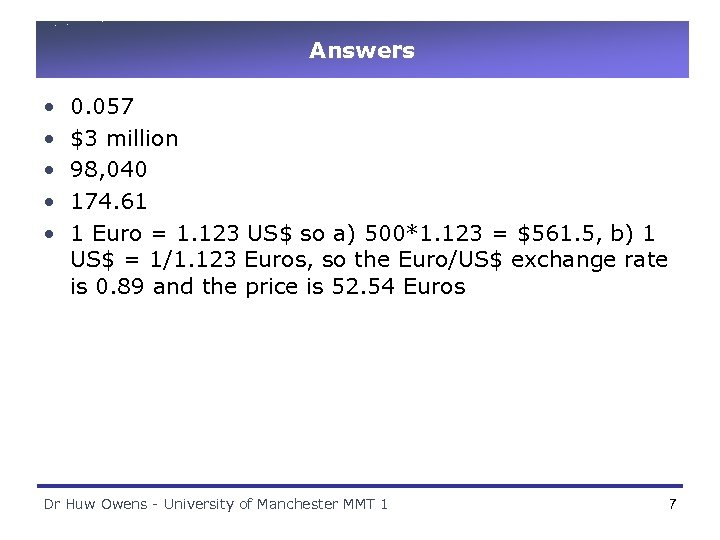Answers • • • 0. 057 \$3 million 98, 040 174. 61 1 Euro = 1. 123 US\$ so a) 500*1. 123 = \$561. 5, b) 1 US\$ = 1/1. 123 Euros, so the Euro/US\$ exchange rate is 0. 89 and the price is 52. 54 Euros Dr Huw Owens - University of Manchester MMT 1 7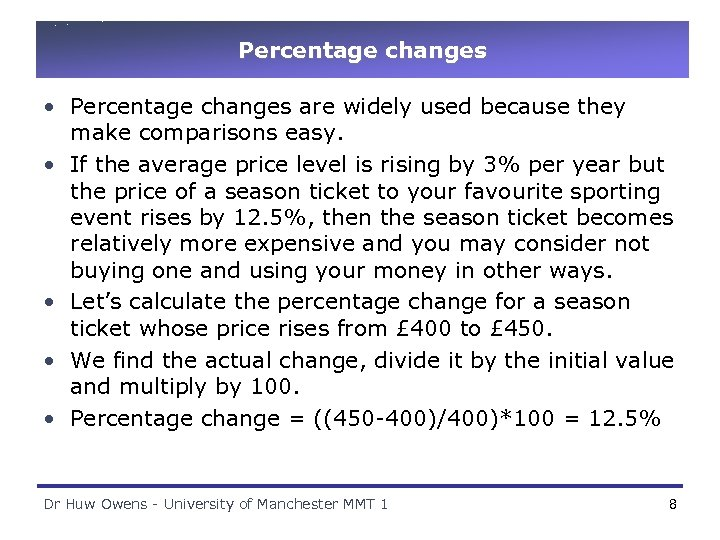Percentage changes • Percentage changes are widely used because they make comparisons easy. • If the average price level is rising by 3% per year but the price of a season ticket to your favourite sporting event rises by 12. 5%, then the season ticket becomes relatively more expensive and you may consider not buying one and using your money in other ways. • Let’s calculate the percentage change for a season ticket whose price rises from £ 400 to £ 450. • We find the actual change, divide it by the initial value and multiply by 100. • Percentage change = ((450 -400)/400)*100 = 12. 5% Dr Huw Owens - University of Manchester MMT 1 8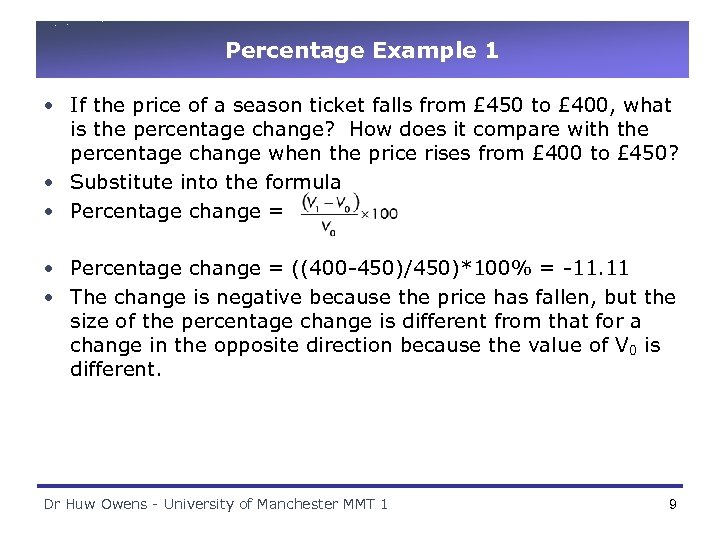Percentage Example 1 • If the price of a season ticket falls from £ 450 to £ 400, what is the percentage change? How does it compare with the percentage change when the price rises from £ 400 to £ 450? • Substitute into the formula • Percentage change = ((400 -450)/450)*100% = -11. 11 • The change is negative because the price has fallen, but the size of the percentage change is different from that for a change in the opposite direction because the value of V 0 is different. Dr Huw Owens - University of Manchester MMT 1 9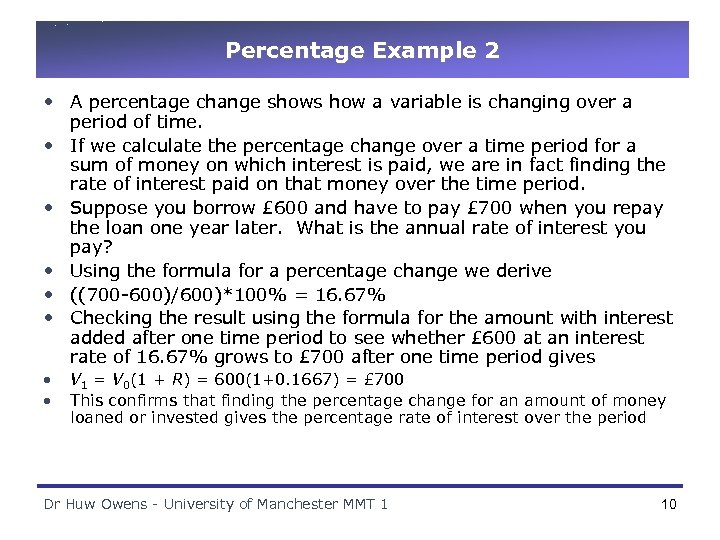Percentage Example 2 • A percentage change shows how a variable is changing over a period of time. • If we calculate the percentage change over a time period for a sum of money on which interest is paid, we are in fact finding the rate of interest paid on that money over the time period. • Suppose you borrow £ 600 and have to pay £ 700 when you repay the loan one year later. What is the annual rate of interest you pay? • Using the formula for a percentage change we derive • ((700 -600)/600)*100% = 16. 67% • Checking the result using the formula for the amount with interest added after one time period to see whether £ 600 at an interest rate of 16. 67% grows to £ 700 after one time period gives • • V 1 = V 0(1 + R) = 600(1+0. 1667) = £ 700 This confirms that finding the percentage change for an amount of money loaned or invested gives the percentage rate of interest over the period Dr Huw Owens - University of Manchester MMT 1 10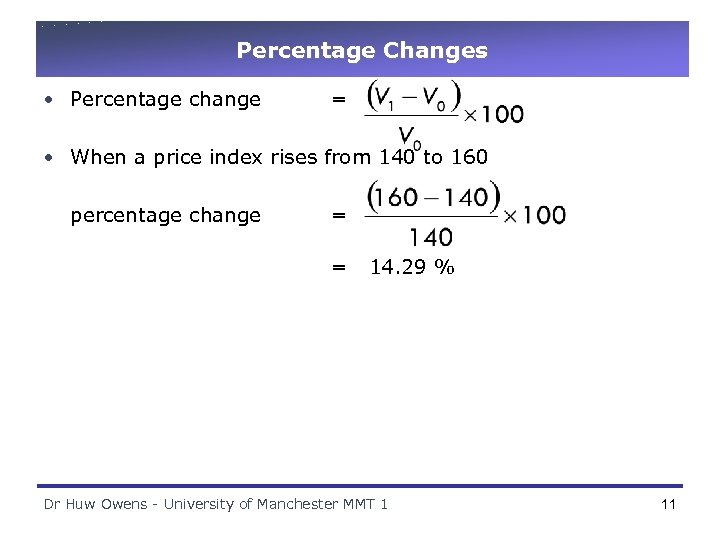Percentage Changes • Percentage change = • When a price index rises from 140 to 160 percentage change = = 14. 29 % Dr Huw Owens - University of Manchester MMT 1 11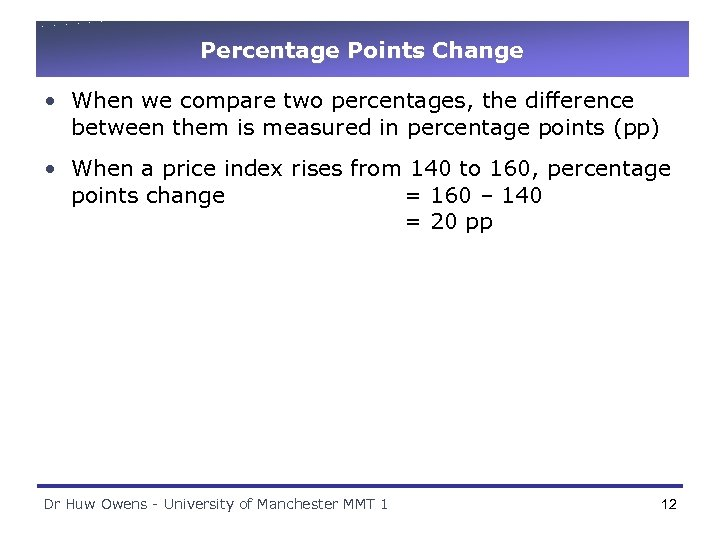Percentage Points Change • When we compare two percentages, the difference between them is measured in percentage points (pp) • When a price index rises from 140 to 160, percentage points change = 160 – 140 = 20 pp Dr Huw Owens - University of Manchester MMT 1 12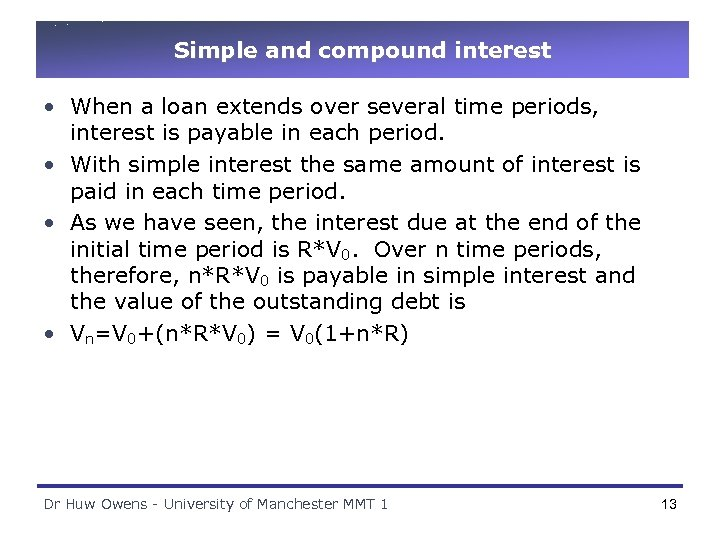Simple and compound interest • When a loan extends over several time periods, interest is payable in each period. • With simple interest the same amount of interest is paid in each time period. • As we have seen, the interest due at the end of the initial time period is R*V 0. Over n time periods, therefore, n*R*V 0 is payable in simple interest and the value of the outstanding debt is • Vn=V 0+(n*R*V 0) = V 0(1+n*R) Dr Huw Owens - University of Manchester MMT 1 13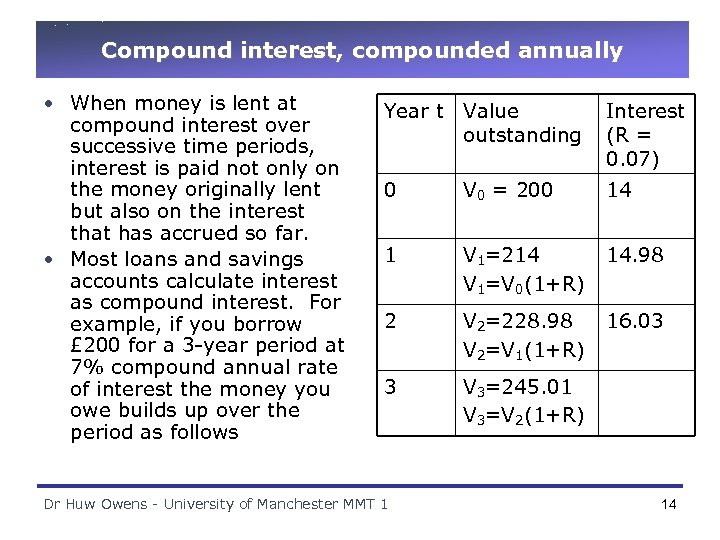Compound interest, compounded annually • When money is lent at compound interest over successive time periods, interest is paid not only on the money originally lent but also on the interest that has accrued so far. • Most loans and savings accounts calculate interest as compound interest. For example, if you borrow £ 200 for a 3 -year period at 7% compound annual rate of interest the money you owe builds up over the period as follows Year t Value outstanding Interest (R = 0. 07) 0 V 0 = 200 14 1 V 1=214 V 1=V 0(1+R) 14. 98 2 V 2=228. 98 V 2=V 1(1+R) 16. 03 3 V 3=245. 01 V 3=V 2(1+R) Dr Huw Owens - University of Manchester MMT 1 14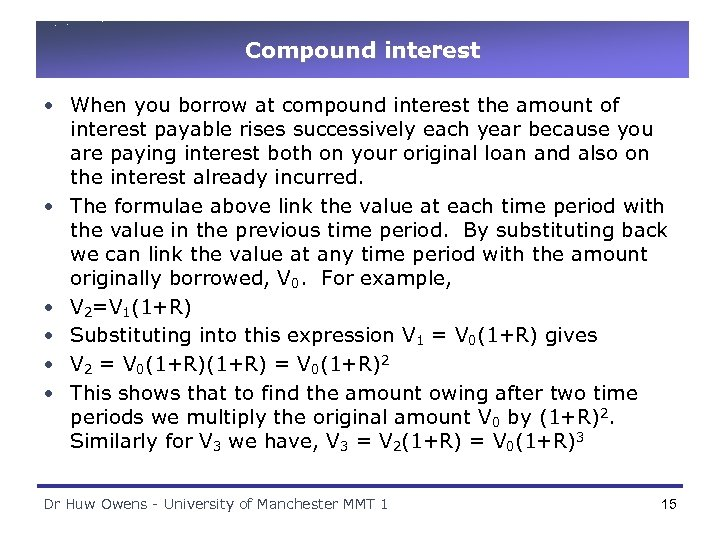Compound interest • When you borrow at compound interest the amount of interest payable rises successively each year because you are paying interest both on your original loan and also on the interest already incurred. • The formulae above link the value at each time period with the value in the previous time period. By substituting back we can link the value at any time period with the amount originally borrowed, V 0. For example, • V 2=V 1(1+R) • Substituting into this expression V 1 = V 0(1+R) gives • V 2 = V 0(1+R)2 • This shows that to find the amount owing after two time periods we multiply the original amount V 0 by (1+R)2. Similarly for V 3 we have, V 3 = V 2(1+R) = V 0(1+R)3 Dr Huw Owens - University of Manchester MMT 1 15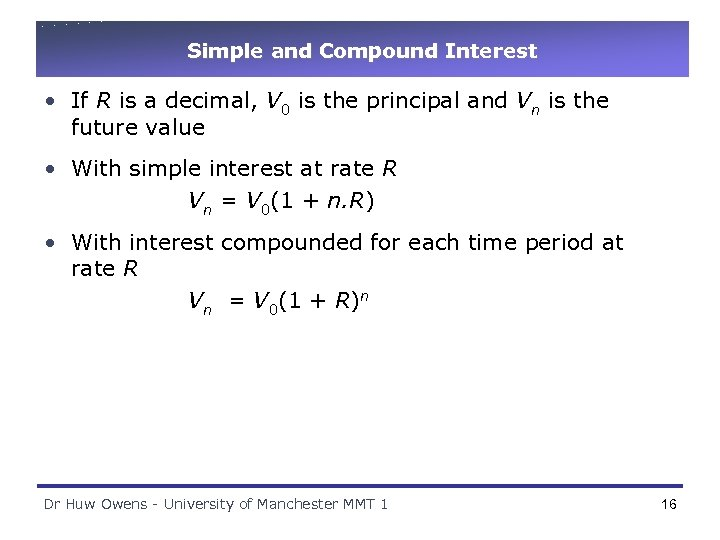Simple and Compound Interest • If R is a decimal, V 0 is the principal and Vn is the future value • With simple interest at rate R Vn = V 0(1 + n. R) • With interest compounded for each time period at rate R Vn = V 0(1 + R)n Dr Huw Owens - University of Manchester MMT 1 16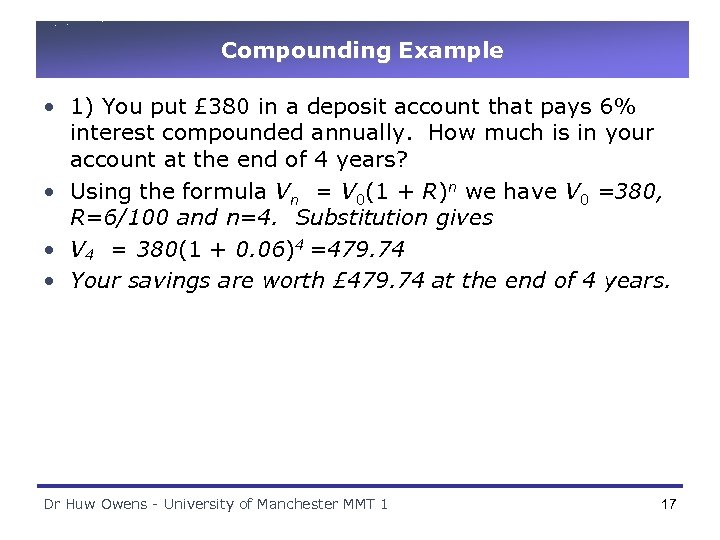Compounding Example • 1) You put £ 380 in a deposit account that pays 6% interest compounded annually. How much is in your account at the end of 4 years? • Using the formula Vn = V 0(1 + R)n we have V 0 =380, R=6/100 and n=4. Substitution gives • V 4 = 380(1 + 0. 06)4 =479. 74 • Your savings are worth £ 479. 74 at the end of 4 years. Dr Huw Owens - University of Manchester MMT 1 17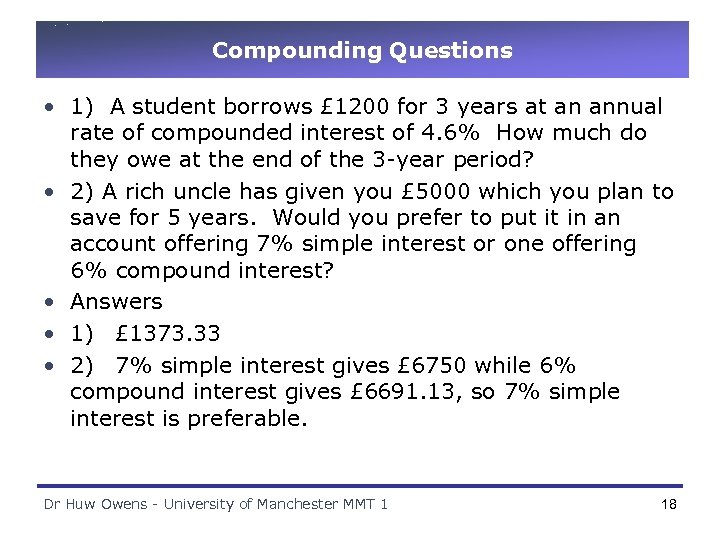Compounding Questions • 1) A student borrows £ 1200 for 3 years at an annual rate of compounded interest of 4. 6% How much do they owe at the end of the 3 -year period? • 2) A rich uncle has given you £ 5000 which you plan to save for 5 years. Would you prefer to put it in an account offering 7% simple interest or one offering 6% compound interest? • Answers • 1) £ 1373. 33 • 2) 7% simple interest gives £ 6750 while 6% compound interest gives £ 6691. 13, so 7% simple interest is preferable. Dr Huw Owens - University of Manchester MMT 1 18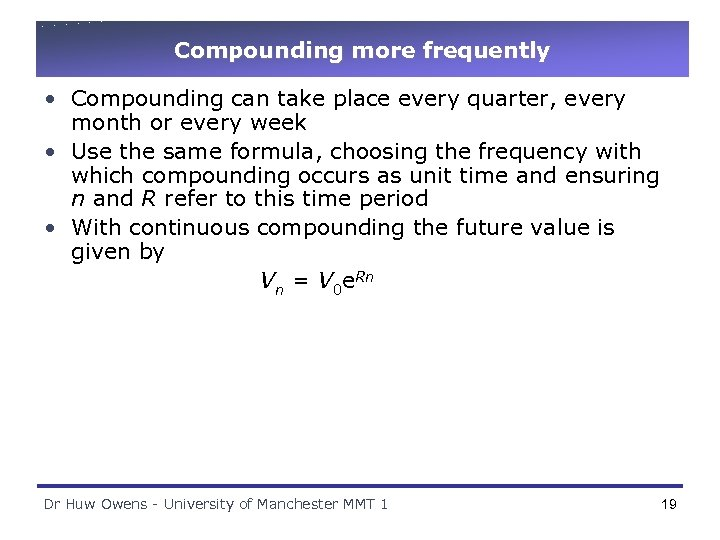Compounding more frequently • Compounding can take place every quarter, every month or every week • Use the same formula, choosing the frequency with which compounding occurs as unit time and ensuring n and R refer to this time period • With continuous compounding the future value is given by Vn = V 0 e. Rn Dr Huw Owens - University of Manchester MMT 1 19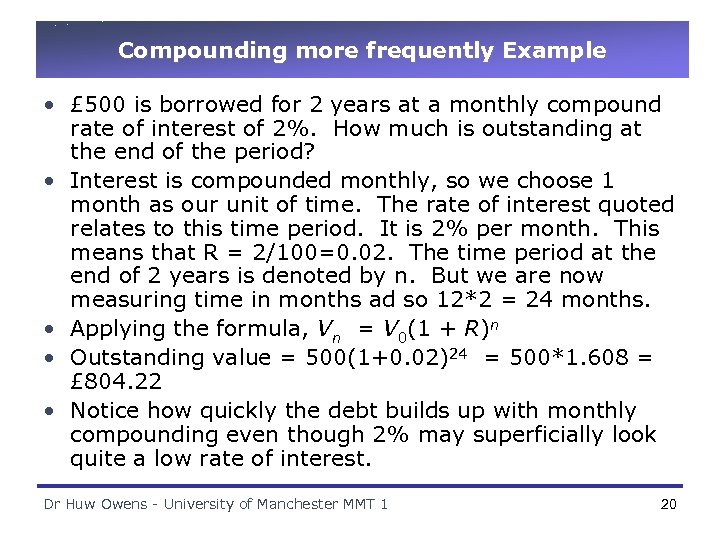Compounding more frequently Example • £ 500 is borrowed for 2 years at a monthly compound rate of interest of 2%. How much is outstanding at the end of the period? • Interest is compounded monthly, so we choose 1 month as our unit of time. The rate of interest quoted relates to this time period. It is 2% per month. This means that R = 2/100=0. 02. The time period at the end of 2 years is denoted by n. But we are now measuring time in months ad so 12*2 = 24 months. • Applying the formula, Vn = V 0(1 + R)n • Outstanding value = 500(1+0. 02)24 = 500*1. 608 = £ 804. 22 • Notice how quickly the debt builds up with monthly compounding even though 2% may superficially look quite a low rate of interest. Dr Huw Owens - University of Manchester MMT 1 20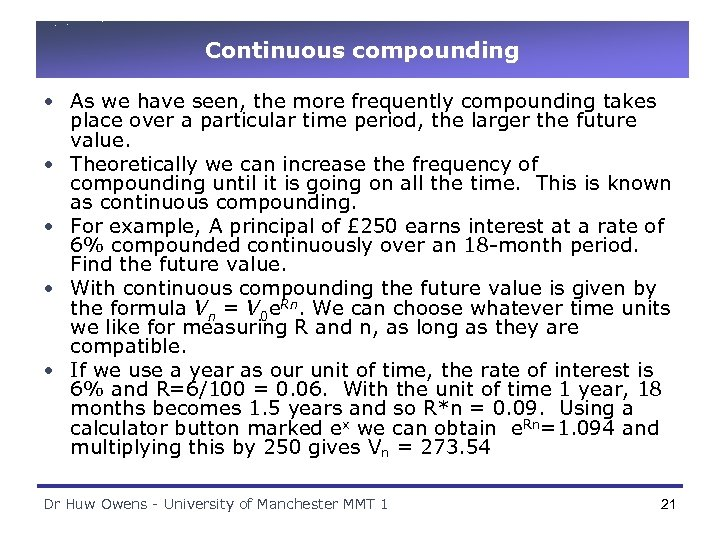Continuous compounding • As we have seen, the more frequently compounding takes place over a particular time period, the larger the future value. • Theoretically we can increase the frequency of compounding until it is going on all the time. This is known as continuous compounding. • For example, A principal of £ 250 earns interest at a rate of 6% compounded continuously over an 18 -month period. Find the future value. • With continuous compounding the future value is given by the formula Vn = V 0 e. Rn. We can choose whatever time units we like for measuring R and n, as long as they are compatible. • If we use a year as our unit of time, the rate of interest is 6% and R=6/100 = 0. 06. With the unit of time 1 year, 18 months becomes 1. 5 years and so R*n = 0. 09. Using a calculator button marked ex we can obtain e. Rn=1. 094 and multiplying this by 250 gives Vn = 273. 54 Dr Huw Owens - University of Manchester MMT 1 21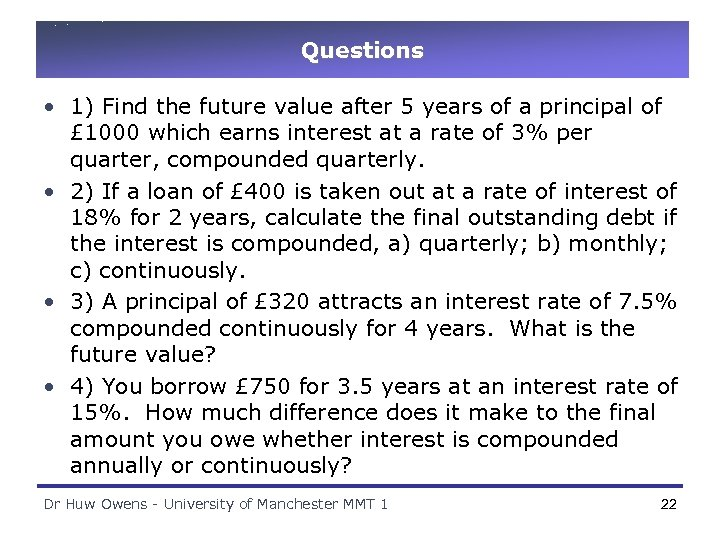Questions • 1) Find the future value after 5 years of a principal of £ 1000 which earns interest at a rate of 3% per quarter, compounded quarterly. • 2) If a loan of £ 400 is taken out at a rate of interest of 18% for 2 years, calculate the final outstanding debt if the interest is compounded, a) quarterly; b) monthly; c) continuously. • 3) A principal of £ 320 attracts an interest rate of 7. 5% compounded continuously for 4 years. What is the future value? • 4) You borrow £ 750 for 3. 5 years at an interest rate of 15%. How much difference does it make to the final amount you owe whether interest is compounded annually or continuously? Dr Huw Owens - University of Manchester MMT 1 22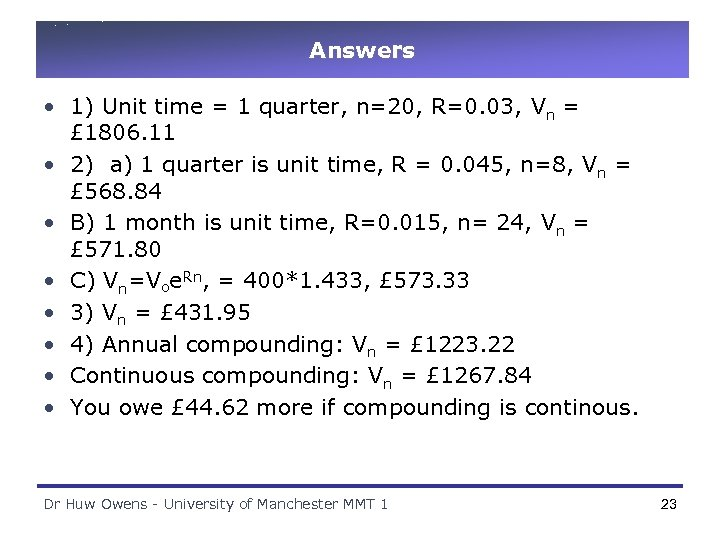Answers • 1) Unit time = 1 quarter, n=20, R=0. 03, Vn = £ 1806. 11 • 2) a) 1 quarter is unit time, R = 0. 045, n=8, Vn = £ 568. 84 • B) 1 month is unit time, R=0. 015, n= 24, Vn = £ 571. 80 • C) Vn=Voe. Rn, = 400*1. 433, £ 573. 33 • 3) Vn = £ 431. 95 • 4) Annual compounding: Vn = £ 1223. 22 • Continuous compounding: Vn = £ 1267. 84 • You owe £ 44. 62 more if compounding is continous. Dr Huw Owens - University of Manchester MMT 1 23